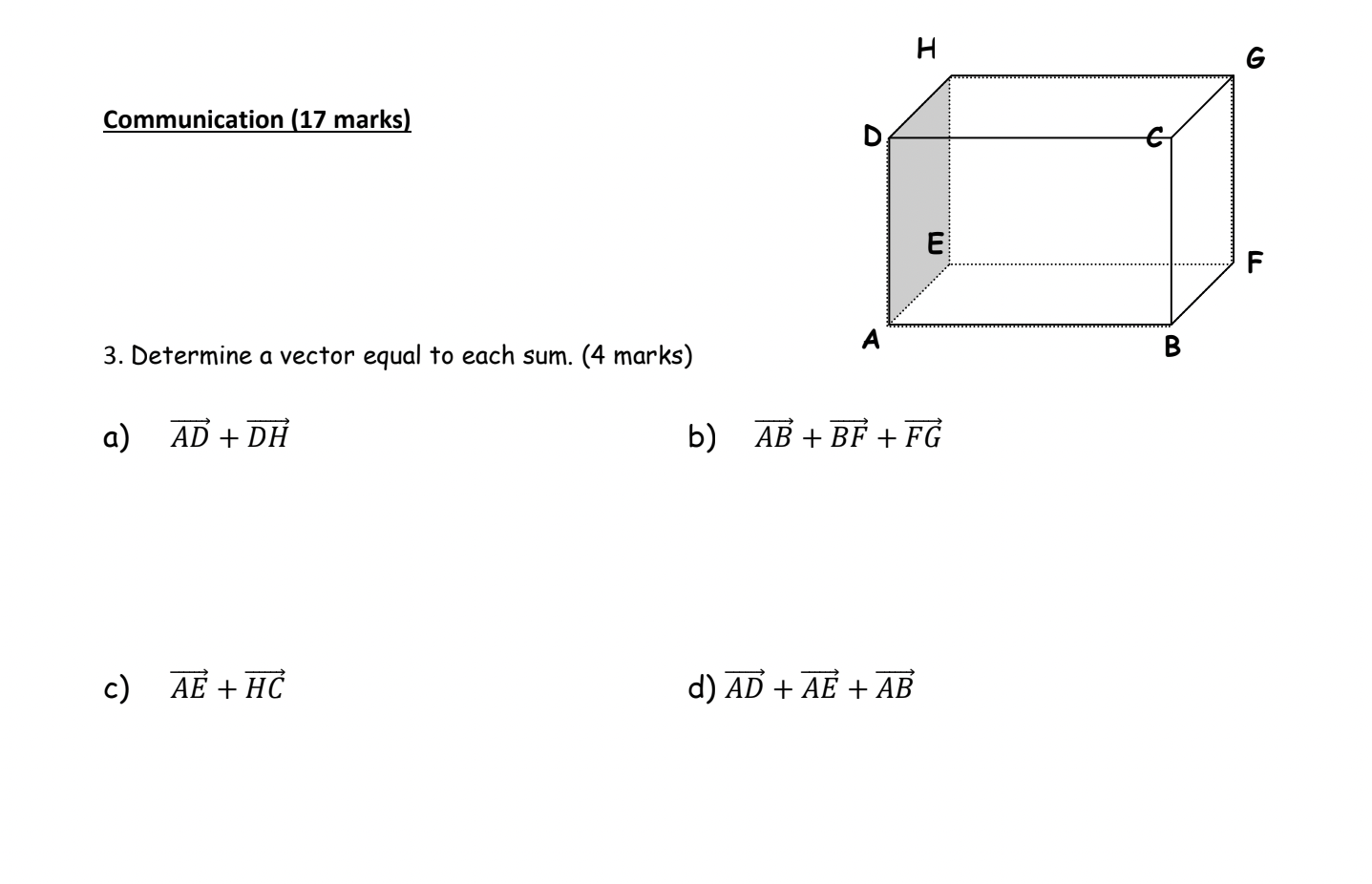# Question Solved1 AnswerPlease answer as soon as possible! thank you very much Communication (17 marks) 3. Determine a vector equal to each sum. (4 marks) a) AD + DH c) AE + HC H E b) AB + BF + FG d) AD + AE + AB S FTranscribed Image Text: Communication (17 marks) 3. Determine a vector equal to each sum. (4 marks) a) AD + DH c) AE + HC H E b) AB + BF + FG d) AD + AE + AB S F
More
Transcribed Image Text: Communication (17 marks) 3. Determine a vector equal to each sum. (4 marks) a) AD + DH c) AE + HC H E b) AB + BF + FG d) AD + AE + AB S F